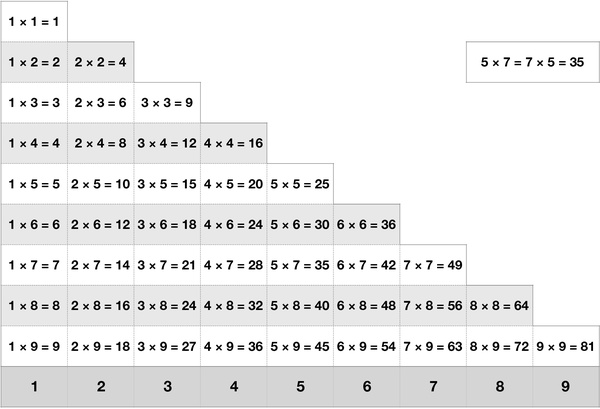# Chinese Multiplication Table 9 by 9In mathematics, a multiplication table or, a times table, is a mathematical table used to define a multiplication operation for numbers. The Chinese multiplication table consists of eighty-one multiplication short equations, making it easy for children to learn by heart. A shorter version of the table consists of only forty-five terms, with duplicates eliminated by applying the commutative property. The multiplication table was traditionally taught as an essential part of elementary arithmetic around the world, as it lays the foundation for arithmetic operations with base-ten numbers.

This worksheet contains a chart of 45 multiplication results of factors from 1 to 9. Memorizing the multiplication table is an important skill for learning mathematics. Once kids master this skill, they could do calculations quickly and accurately without using calculators. It is better to introduce the multiplication table to kids as soon as they start learning numbers and counting.